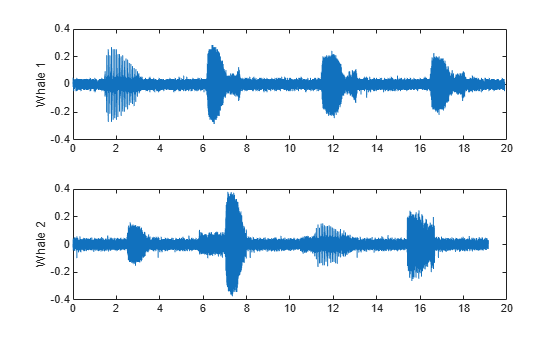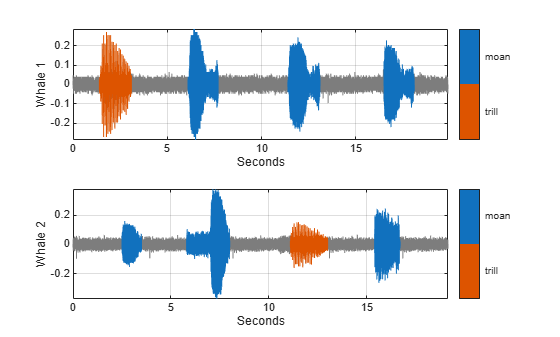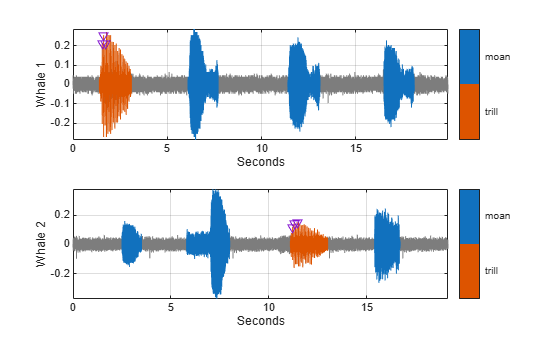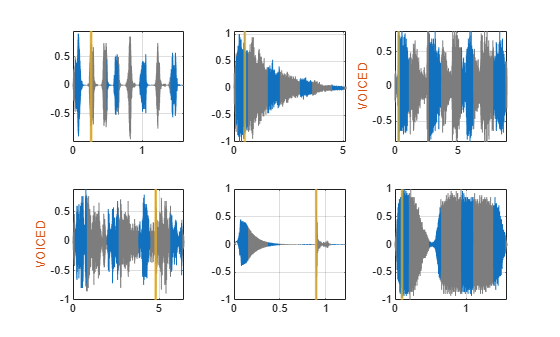signalLabelDefinition

Create signal label definition

Description

Use signalLabelDefinition to create signal label definitions for data sets. The labels can correspond to attributes, regions, or points of interest. Use a vector of signalLabelDefinition objects to create a labeledSignalSet.

Creation

Description

sld = signalLabelDefinition(name) creates a signal label definition object, sld, with the Name property set to name and other properties set to default values.

example

sld = signalLabelDefinition(name,Name,Value) sets Properties using name-value pairs. You can specify multiple name-value pairs. Enclose each property name in quotes.

Input Arguments

expand all

Label name, specified as a character vector or string scalar.

Data Types: char | string

Properties

expand all

Name of label, specified as a character vector or string scalar.

Data Types: char | string

Type of label, specified as one of the following:

• 'attribute' — Define signal characteristics.

• 'roi' — Define signal characteristics over regions of interest.

• 'point' — Define signal characteristics over points of interest.

Data Types: char | string

Data type of label, specified as 'logical', 'categorical', 'numeric', 'string', 'table', or 'timetable'. Use the Categories property to specify the array of categories when this property is set to 'categorical'.

Data Types: char | string

Label category names, specified as a string array or a cell array of character vectors. The array must have unique elements. This property applies only when the LabelDataType property is set to 'categorical'.

Example: 'LabelDataType','categorical','Categories',["apple","orange"]

Data Types: char | string

Data type of ROI limits, specified as either 'double' or 'duration'. This property applies only when LabelType is set to 'roi'.

Data Types: char | string

Data type of point locations, specified as either 'double' or 'duration'. This property applies only when LabelType is set to 'point'.

Data Types: char | string

Validation function, specified as a function handle and used when setting label values in a labeledSignalSet object. This property applies only when LabelDataType is set to 'categorical', 'logical', 'numeric', 'table', or 'timetable'. If not specified, the function checks only that its input values are of the correct data type. If LabelDataType is set to 'categorical', the function checks that the input is one of the values specified using Categories. The function takes an input value and returns true if the value is valid and false if the value is invalid.

Example: 'LabelDataType','numeric','DefaultValue',1,'ValidationFunction',@(x)x<2

Data Types: function_handle

Default value of label, specified as a value of the type specified using LabelDataType. If LabelDataType is set to 'categorical', then DefaultValue must be one of the values specified using Categories.

Example: 'LabelDataType','categorical','Categories',["apple","orange"],'DefaultValue',"apple"

Data Types: char | double | logical | string | table

Label description, specified as a character vector or string scalar.

Example: 'Description','Patient is asleep'

Data Types: char | string

Label tag identifier, specified as a character vector or string scalar. Use this property to identify the same label in a larger labeling scheme or public labeling set.

Example: 'Tag','Peak1'

Data Types: char | string

Array of sublabels, specified as a signal label definition object. To specify more than one sublabel, set this property to a vector of signal label definition objects. Use this property to create a relationship between a parent label and its children.

Note

Sublabels cannot have sublabels.

Example: 'Sublabels',[signalLabelDefinition("negative"),signalLabelDefinition("positive")]

Object Functions

 labelDefinitionsHierarchy Get hierarchical list of label and sublabel names labelDefinitionsSummary Get summary table of signal label definitions

Examples

collapse all

Consider a set of whale sound recordings. The recorded whale sounds consist of trills and moans. Trills sound like series of clicks. Moans are low-frequency cries similar to the sound made by a ship's horn. You want to look at each signal and label it to identify the whale type, the trill regions, and the moan regions. For each trill region, you also want to label the signal peaks higher than a certain threshold.

Signal Label Definitions

Define an attribute label to store whale types. The possible categories are blue whale, humpback whale, and white whale.

dWhaleType = signalLabelDefinition('WhaleType',...
'LabelType','attribute',...
'LabelDataType','categorical',...
'Categories',{'blue','humpback','white'},...
'Description','Whale type');

Define a region-of-interest (ROI) label to capture moan regions. Define another ROI label to capture trill regions.

dMoans = signalLabelDefinition('MoanRegions',...
'LabelType','roi',...
'LabelDataType','logical',...
'Description','Regions where moans occur');

dTrills = signalLabelDefinition('TrillRegions',...
'LabelType','roi',...
'LabelDataType','logical',...
'Description','Regions where trills occur');

Finally, define a point label to capture the trill peaks. Set this label as a sublabel of the dTrills definition.

dTrillPeaks = signalLabelDefinition('TrillPeaks',...
'LabelType','point',...
'LabelDataType','numeric',...
'Description','Trill peaks');

dTrills.Sublabels = dTrillPeaks;

Labeled Signal Set

Create a labeledSignalSet with the whale signals and the label definitions. Add label values to identify the whale type, the moan and trill regions, and the peaks of the trills.

lbldefs = [dWhaleType dMoans dTrills];

lss = labeledSignalSet({whale1 whale2},lbldefs,'MemberNames',{'Whale1','Whale2'}, ...
'SampleRate',Fs,'Description','Characterize whale song regions');

Visualize the label hierarchy and label properties using labelDefinitionsHierarchy and labelDefinitionsSummary.

labelDefinitionsHierarchy(lss)
ans =
'WhaleType
Sublabels: []
MoanRegions
Sublabels: []
TrillRegions
Sublabels: TrillPeaks
'

labelDefinitionsSummary(lss)
ans=3×9 table
LabelName        LabelType     LabelDataType     Categories     ValidationFunction    DefaultValue             Sublabels             Tag            Description
______________    ___________    _____________    ____________    __________________    ____________    ___________________________    ___    ____________________________

"WhaleType"       "attribute"    "categorical"    {3x1 string}       {["N/A"   ]}       {0x0 double}    {0x0 double               }    ""     "Whale type"
"MoanRegions"     "roi"          "logical"        {["N/A"   ]}       {0x0 double}       {0x0 double}    {0x0 double               }    ""     "Regions where moans occur"
"TrillRegions"    "roi"          "logical"        {["N/A"   ]}       {0x0 double}       {0x0 double}    {1x1 signalLabelDefinition}    ""     "Regions where trills occur"

The signals in the loaded data correspond to songs of two blue whales. Set the 'WhaleType' values for both signals.

setLabelValue(lss,1,'WhaleType','blue');
setLabelValue(lss,2,'WhaleType','blue');

Visualize the 'Labels' property. The table has the newly added 'WhaleType' values for both signals.

lss.Labels
ans=2×3 table
WhaleType    MoanRegions    TrillRegions
_________    ___________    ____________

Whale1      blue       {0x2 table}    {0x3 table}
Whale2      blue       {0x2 table}    {0x3 table}

Visualize Region Labels

Visualize the whale songs to identify the trill and moan regions.

subplot(2,1,1)
plot((0:length(whale1)-1)/Fs,whale1)
ylabel('Whale 1')

subplot(2,1,2)
plot((0:length(whale2)-1)/Fs,whale2)
ylabel('Whale 2')Moan regions are sustained low-frequency wails.

• whale1 has moans centered at about 7 seconds, 12 seconds, and 17 seconds.

• whale2 has moans centered at about 3 seconds, 7 seconds, and 16 seconds.

Add the moan regions to the labeled set. Specify the ROI limits in seconds and the label values.

moanRegionsWhale1 = [6.1 7.7; 11.4 13.1; 16.5 18.1];
mrsz1 = [size(moanRegionsWhale1,1) 1];
setLabelValue(lss,1,'MoanRegions',moanRegionsWhale1,true(mrsz1));

moanRegionsWhale2 = [2.5 3.5; 5.8 8; 15.4 16.7];
mrsz2 = [size(moanRegionsWhale2,1) 1];
setLabelValue(lss,2,'MoanRegions',moanRegionsWhale2,true(mrsz2));

Trill regions have distinct bursts of sound punctuated by silence.

• whale1 has a trill centered at about 2 seconds.

• whale2 has a trill centered at about 12 seconds.

Add the trill regions to the labeled set.

trillRegionWhale1 = [1.4 3.1];
trsz1 = [size(trillRegionWhale1,1) 1];
setLabelValue(lss,1,'TrillRegions',trillRegionWhale1,true(trsz1));

trillRegionWhale2 = [11.1 13];
trsz2 = [size(trillRegionWhale1,1) 1];
setLabelValue(lss,2,'TrillRegions',trillRegionWhale2,true(trsz2));

Create a signalMask (Signal Processing Toolbox) object for each whale song and use it to visualize and label the different regions. For better visualization, change the label values from logical to categorical.

mr1 = getLabelValues(lss,1,'MoanRegions');
mr1.Value = categorical(repmat("moan",mrsz1));
tr1 = getLabelValues(lss,1,'TrillRegions');
tr1.Value = categorical(repmat("trill",trsz1));

subplot(2,1,1)
plotsigroi(msk1,whale1)
ylabel('Whale 1')
hold on

mr2 = getLabelValues(lss,2,'MoanRegions');
mr2.Value = categorical(repmat("moan",mrsz2));
tr2 = getLabelValues(lss,2,'TrillRegions');
tr2.Value = categorical(repmat("trill",trsz2));

subplot(2,1,2)
plotsigroi(msk2,whale2)
ylabel('Whale 2')
hold onVisualize Point Labels

Label three peaks for each trill region. For point labels, you specify the point locations and the label values. In this example, the point locations are in seconds.

peakLocsWhale1 = [1.553 1.626 1.7];
peakValsWhale1 = [0.211 0.254 0.211];

setLabelValue(lss,1,{'TrillRegions','TrillPeaks'}, ...
peakLocsWhale1,peakValsWhale1,'LabelRowIndex',1);

subplot(2,1,1)
plot(peakLocsWhale1,peakValsWhale1,'v')
hold off

peakLocsWhale2 = [11.214 11.288 11.437];
peakValsWhale2 = [0.119 0.14 0.15];

setLabelValue(lss,2,{'TrillRegions','TrillPeaks'}, ...
peakLocsWhale2,peakValsWhale2,'LabelRowIndex',1);

subplot(2,1,2)
plot(peakLocsWhale2,peakValsWhale2,'v')
hold offExplore Label Values

Explore the label values using getLabelValues.

getLabelValues(lss)
ans=2×3 table
WhaleType    MoanRegions    TrillRegions
_________    ___________    ____________

Whale1      blue       {3x2 table}    {1x3 table}
Whale2      blue       {3x2 table}    {1x3 table}

Retrieve the moan regions for the first member of the labeled set.

getLabelValues(lss,1,'MoanRegions')
ans=3×2 table
ROILimits      Value
____________    _____

6.1     7.7    {}
11.4    13.1    {}
16.5    18.1    {}

Use a second output argument to list the sublabels of a label.

[value,valueWithSublabel] = getLabelValues(lss,1,'TrillRegions')
value=1×2 table
ROILimits     Value
__________    _____

1.4    3.1    {}

valueWithSublabel=1×3 table
ROILimits     Value     Sublabels
TrillPeaks
__________    _____    ___________

1.4    3.1    {}    {3x2 table}

To retrieve the values in a sublabel, express the label name as a two-element array.

getLabelValues(lss,1,{'TrillRegions','TrillPeaks'})
ans=3×2 table
Location      Value
________    __________

1.553      {[0.2110]}
1.626      {[0.2540]}
1.7      {[0.2110]}

Find the value of the third trill peak corresponding to the second member of the set.

getLabelValues(lss,2,{'TrillRegions','TrillPeaks'}, ...
'LabelRowIndex',1,'SublabelRowIndex',3)
ans=1×2 table
Location      Value
________    __________

11.437     {[0.1500]}

Specify the path to a set of audio signals included as MAT-files with MATLAB®. Each file contains a signal variable and a sample rate. List the names of the files.

folder = fullfile(matlabroot,"toolbox","matlab","audiovideo");
lst = dir(append(folder,"/*.mat"));
nms = {lst(:).name}'
nms = 7x1 cell
{'chirp.mat'   }
{'gong.mat'    }
{'handel.mat'  }
{'laughter.mat'}
{'mtlb.mat'    }
{'splat.mat'   }
{'train.mat'   }

Create a signal datastore that points to the specified folder. Set the sample rate variable name to Fs, which is common to all files. Generate a subset of the datastore that excludes the file mtlb.mat. Use the subset datastore as the source for a labeledSignalSet object.

sds = signalDatastore(folder,"SampleRateVariableName","Fs");
sds = subset(sds,~strcmp(nms,"mtlb.mat"));
lss = labeledSignalSet(sds);

Create three label definitions to label the signals:

• Define a logical attribute label that is true for signals that contain human voices.

• Define a numeric point label that marks the location and amplitude of the maximum of each signal.

• Define a categorical region-of-interest (ROI) label to pick out nonoverlapping, uniform-length random regions of each signal.

Add the signal label definitions to the labeled signal set.

vc = signalLabelDefinition("Voice",'LabelType','attribute', ...
'LabelDataType','logical','DefaultValue',false);
mx = signalLabelDefinition("Maximum",'LabelType','point', ...
'LabelDataType','numeric');
rs = signalLabelDefinition("RanROI",'LabelType','ROI', ...
'LabelDataType','categorical','Categories',["ROI" "other"]);

Label the signals:

• Label 'handel.mat' and 'laughter.mat' as having human voices.

• Use the islocalmax function to find the maximum of each signal. Label its location and value.

• Use the randROI function to generate as many regions of length N/10 samples as can fit in a signal of length N given a minimum separation of N/6 samples between regions. Label their locations and assign them to the ROI category.

When labeling points and regions, convert sample values to time values. Subtract 1 to account for MATLAB® array indexing and divide by the sample rate.

kj = 1;
while hasdata(sds)

fs = info.SampleRate;

[~,fn] = fileparts(info.FileName);
if fn=="handel" || fn=="laughter"
setLabelValue(lss,kj,"Voice",true)
end

xm = find(islocalmax(sig,'MaxNumExtrema',1));
setLabelValue(lss,kj,"Maximum",(xm-1)/fs,sig(xm))

N = length(sig);
rois = randROI(N,round(N/10),round(N/6));
setLabelValue(lss,kj,"RanROI",(rois-1)/fs,repelem("ROI",size(rois,1)))

kj = kj+1;

end

Verify that only two signals contain voices.

countLabelValues(lss,"Voice")
ans=2×3 table
Voice    Count    Percent
_____    _____    _______

false      4      66.667
true       2      33.333

Verify that two signals have a maximum amplitude of 1.

countLabelValues(lss,"Maximum")
ans=5×4 table
Maximum            Count    Percent    MemberCount
______________________    _____    _______    ___________

0.80000000000000004441      1      16.667          1
0.89113331915798421612      1      16.667          1
0.94730769230769229505      1      16.667          1
1                           2      33.333          2
1.0575668990330560071       1      16.667          1

Verify that each signal has four nonoverlapping random regions of interest.

countLabelValues(lss,"RanROI")
ans=2×4 table
RanROI    Count    Percent    MemberCount
______    _____    _______    ___________

ROI        24        100           6
other       0          0           0

Create two datastores with the data in the labeled signal set:

• The signalDatastore (Signal Processing Toolbox) object sd contains the signal data.

• The arrayDatastore object ld contains the labeling information. Specify that you want to include the information corresponding to all the labels you created.

[sd,ld] = createDatastores(lss,["Voice" "RanROI" "Maximum"]);

Use the information in the datastores to plot the signals and display their labels.

• Use a signalMask (Signal Processing Toolbox) object to highlight the regions of interest in blue.

• Plot yellow lines to mark the locations of the maxima.

• Add a red axis label to the signals that contain human voices.

tiledlayout flow

while hasdata(sd)

nexttile

plotsigroi(msk,sg)
colorbar off
xlabel('')

xline(lbls{:}.Maximum{:}.Location, ...
'LineWidth',2,'Color','#EDB120')

if lbls{:}.Voice{:}
ylabel('VOICED','Color','#D95319')
end

endfunction roilims = randROI(N,wid,sep)

num = floor((N+sep)/(wid+sep));
hq = histcounts(randi(num+1,1,N-num*wid-(num-1)*sep),(1:num+2)-1/2);
roilims = (1 + (0:num-1)*(wid+sep) + cumsum(hq(1:num)))' + [0 wid-1];

end

Objects

Introduced in R2018b

Wavelet Toolbox Documentation평가판 신청